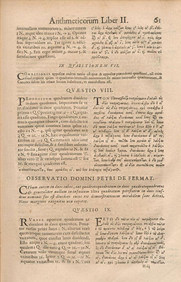The 1670 edition of Diophantus’ Arithmetica includes Fermat’s commentary, particularly his ‘Last Theorem’.

When you are at school it might seem like every problem in maths has already been solved by somebody else. There is always an answer at the back of a text book or a solution ready to be given by your teacher. In fact there are still many unsolved mathematical problems waiting to be unraveled by the next generation of mathematicians.

Sometimes mathematical problems can remain unsolved for hundreds of years, and people wonder whether a proof is even possible. This was the case with Fermat’s last theorem, a theorem first written in 1637 by Pierre de Fermat, a French mathematician.

 Fermat’s last theorem The theorem states that when n is a positive whole number bigger than 2, no other positive whole numbers a, b and c can be found so that an+bn=cn. (If n=2, there are lots of values for a, b and c which work. In fact we are looking at Pythagoras’ Theorem!)

In 1995 a mathematician Andrew Wiles published a proof for Fermat’s last theorem, showing that even famous historical unsolved theorems can be proved in the modern day.

The more you study mathematics, the more you will appreciate that mathematics is a vast subject, with always new problems to be solved.

There are however some particularly famous problems which still need solutions. To celebrate the millennium, the Clay Mathematics Institute collected seven of these together and offered a prize of \$1,000,000 to anyone who could provide a solution to any one of the seven problems.

 Millennium Prize Problems 1. Poincaré Conjecture 2. P versus NP 3. Hodge Conjecture 4. Riemann Hypothesis 5. Yang-Mills existence and mass gap 6. Navier-Stokes existence and smoothness 7. Birch and Swinnerton-Dyer Conjecture

Only one of the seven Millennium Prize problems has been solved so far. In 2010 Russian mathematician Grigori Perelman proved the Poincaré Conjecture which is a problem in Topology, a type of geometry. Perelman decided however not to accept the prize, so as of the moment no Millennium Prizes have actually been awarded by the Clay Mathematics Institute.

 There are currently thousands of professional mathematicians working in the UK, both in universities and also in industry and business. If you are good at mathematics at school, then you may well be suited to a career in research. To pursue this career you should try and learn as much mathematics as possible – choosing both A-level Mathematics and Further Mathematics if you can. Try also to hone your problem solving skills – perhaps by solving puzzles or entering mathematical competitions. You never know – in the future you could be someone who helps to solve another of the Millennium Prize Problems.

Article by Hazel Lewis